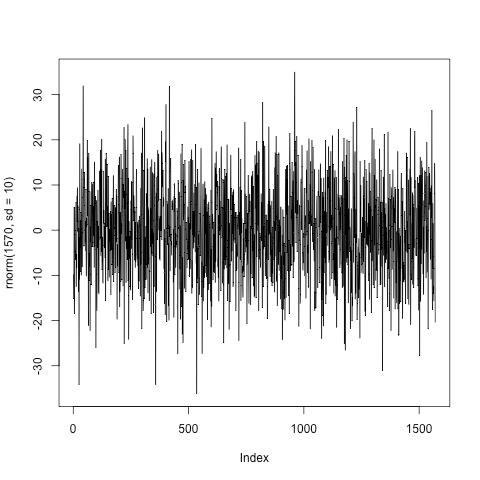Want to share your content on R-bloggers? click here if you have a blog, or here if you don't.

I was re-reading Michael Murray’s explanation of cointegration:

and marvelling at the calculus.

Of course it’s not any subtraction. It’s subtracting a function from a shifted version of itself. Still doesn’t sound like a universal revolution.

(But of course the observation that the lagged first-difference will be zero around an extremum (turning point), along with symbolic formulæ the (infinitesimal) first-differences of a function, made a decent splash.)

Jeff Ryan wrote some R functions that make it easy to first-difference financial time series.Here’s how to do the first differences of Goldman Sachs’ share price:

```require(quantmod)
getSymbols("GS")
plot(  gs - lag(gs)  )
```Look how much more structured the result is! Now all of the numbers are within a fairly narrow band. With `length(gs)` I found 1570 observations. Here are 1570 random normals `plot(rnorm(1570, sd=10), type="l")` for comparison:Not perfectly similar, but very close!

Looking at the first differences compared to a Gaussian brings out what’s different between public equity markets and a random walk. What sticks out to me is the vol leaping up aperiodically in the \$GS time series.

I think I got even a little closer with drawing the stdev’s from a Poisson process `plot(rnorm(1570, sd=rpois(1570, lambda=5)), type="l")`but I’ll end there with the graphical futzing.

What’s really amazing to me is how much difference a subtraction makes.